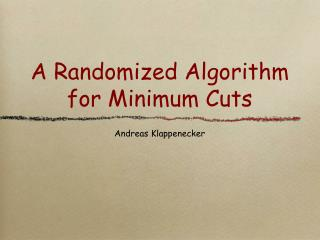Download PresentationA Randomized Algorithm for Minimum Cuts

# A Randomized Algorithm for Minimum Cuts - PowerPoint PPT Presentation

A Randomized Algorithm for Minimum Cuts. Andreas Klappenecker. Connected Multigraphs. Cut. Size of a Cut. The size of a cut is given by the number of edges crossing the cut. Our goal is to determine the minimum size of a cut in a multigraph. Edge Contraction.I am the owner, or an agent authorized to act on behalf of the owner, of the copyrighted work described.
Download Presentation## A Randomized Algorithm for Minimum Cuts

An Image/Link below is provided (as is) to download presentation

Download Policy: Content on the Website is provided to you AS IS for your information and personal use and may not be sold / licensed / shared on other websites without getting consent from its author.While downloading, if for some reason you are not able to download a presentation, the publisher may have deleted the file from their server.

- - - - - - - - - - - - - - - - - - - - - - - - - - E N D - - - - - - - - - - - - - - - - - - - - - - - - - -
Presentation Transcript
1. A Randomized Algorithm for Minimum Cuts • Andreas Klappenecker

2. Connected Multigraphs

3. Cut

4. Size of a Cut • The size of a cut is given by the number of edges crossing the cut. • Our goal is to determine the minimum size of a cut in a multigraph.

5. Edge Contraction

6. Cuts in the Contracted Multigraph

7. Randomized Algorithm

8. Basic Idea • The algorithm Contract selects uniformly at random one of the remaining edges and contracts this edge until two vertices remain. • The cut determined by these two vertices contains precisely the edges that have not been contracted. • Counting the number of edges between the two vertices gives an upper bound on the minimum size of a cut.

9. Two Example Runs Contract {E,F} Contract {D,F} Contract {C,D} Contract {B,D}

10. Possible Errors • The examples show some unsettling property of the algorithm Contract: It does not always return the size of a minimum cut. • How likely is it that the algorithm produces a correct result?

11. Time Complexity

12. Analysis • Let us start with a few simple observations: • Let E1, E2, ..., En be events. Then the definition of a conditional probability yields Pr[E2∩E1] = Pr[E2|E1] Pr[E1]. • Therefore, Pr[E3∩E2∩E1] = Pr[E3|E2∩E1] Pr[E2∩E1] = = Pr[E3|E2∩E1] Pr[E2|E1] Pr[E1] • More generally, • Pr[En∩...∩E2∩E1] = Pr[En|En-1∩...∩E1] Pr[En-1|En-2∩...∩E1] ... Pr[E2|E1] Pr[E1]

13. Prelude • Suppose that a multigraph has just one minimum cut C. • If any edge crossing this cut C is selected by Contract, then the algorithm will not produce the correct size of the minimum cut. • This will be the guiding principle for the analysis. • Our analysis is very pessimistic. If the multigraph has more than one minimum cut (which is typically the case), then a minimum cut will be found with greater probability than our analysis will suggest.

14. Analysis of Contract (1)

15. Analysis of Contract (2)

16. Number of Repetitions • We have shown that the algorithm will • succeed with probability >= 2/n2. • fail with probability <=(1-2/n2). • Since each run is independent, Pr[fail1∩...∩ failn] = Pr[fail1] ... Pr[failn] • Recall from calculus that (1+x/n)n <= exp(x) • Repeating the algorithm Contract n2/2 times, the probability of failure is <= (1-2/n2)(n^2/2)<= e-1. • Therefore, repeating the algorithm Contract 0.5 n2log n times, give a probability of failure Pr[failure] <= e-log n